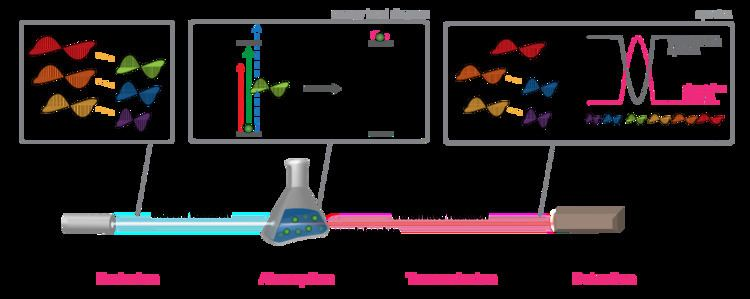Updated on
Covid-19In physics, absorption of electromagnetic radiation is the way in which the energy of a photon is taken up by matter, typically the electrons of an atom. Thus, the electromagnetic energy is transformed into internal energy of the absorber, for example thermal energy. The reduction in intensity of a light wave propagating through a medium by absorption of a part of its photons is often called attenuation. Usually, the absorption of waves does not depend on their intensity (linear absorption), although in certain conditions (usually, in optics), the medium changes its transparency dependently on the intensity of waves going through, and saturable absorption (or nonlinear absorption) occurs.

## Quantifying absorption

There are a number of ways to quantify how quickly and effectively radiation is absorbed in a certain medium, for example:

• The absorption coefficient, and some closely related derived quantities:
• The attenuation coefficient, which is sometimes but not always synonymous with the absorption coefficient
• Molar absorptivity, also called "molar extinction coefficient", which is the absorption coefficient divided by molarity (see also Beer–Lambert law).
• The mass attenuation coefficient, also called "mass extinction coefficient", which is the absorption coefficient divided by density (see also mass attenuation coefficient).
• The absorption cross section and scattering cross-section are closely related to the absorption and attenuation coefficients, respectively.
• "Extinction" in astronomy is equivalent to the attenuation coefficient.
• Penetration depth and skin effect,
• Propagation constant, attenuation constant, phase constant, and complex wavenumber,
• Complex refractive index and extinction coefficient,
• Complex dielectric constant,
• Electrical resistivity and conductivity.
• Absorbance (also called "optical density") and optical depth (also called "optical thickness") are two related measures
• All these quantities measure, at least to some extent, how well a medium absorbs radiation. However, practitioners of different fields and techniques tend to conventionally use different quantities drawn from the list above.

## Measuring absorption

The absorbance of an object quantifies how much of the incident light is absorbed by it (instead of being reflected or refracted). This may be related to other properties of the object through the Beer–Lambert law.

Precise measurements of the absorbance at many wavelengths allow the identification of a substance via absorption spectroscopy, where a sample is illuminated from one side, and the intensity of the light that exits from the sample in every direction is measured. A few examples of absorption are ultraviolet–visible spectroscopy, infrared spectroscopy, and X-ray absorption spectroscopy.

## Applications

Understanding and measuring the absorption of electromagnetic radiation has a variety of applications. Here are a few examples:

• In meteorology and climatology, global and local temperatures depend in part on the absorption of radiation by atmospheric gases (such as in the greenhouse effect) and land and ocean surfaces (see albedo).
• In medicine, X-rays are absorbed to different extents by different tissues (bone in particular), which is the basis for X-ray imaging. For example, see computation of radiowave attenuation in the atmosphere used in satellite link design.
• In chemistry and materials science, because different materials and molecules will absorb radiation to different extents at different frequencies, which allows for material identification.
• In optics, sunglasses, colored filters, dyes, and other such materials are designed specifically with respect to which visible wavelengths they absorb, and in what proportions.
• In biology, photosynthetic organisms require that light of the appropriate wavelengths be absorbed within the active area of chloroplasts, so that the light energy can be converted into chemical energy within sugars and other molecules.
• ## References

Similar Topics
Biyer Lagna